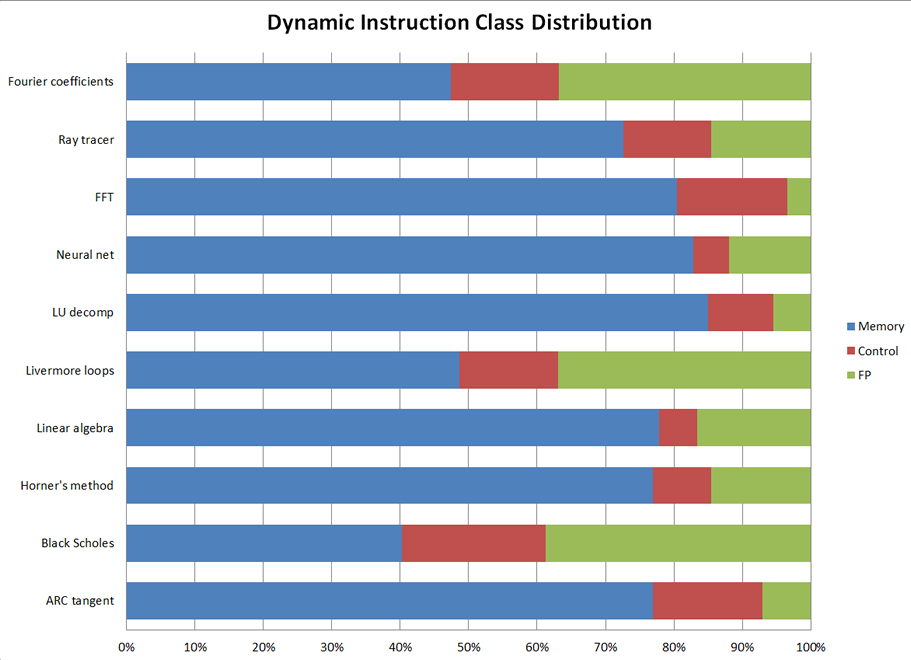### Introduction to the EEMBC FPMark™ Floating-Point Benchmark Suite

FPMark is the embedded industry's first floating-point benchmark software suite. Floating point arithmetic is appearing in many embedded applications such as audio, DSP/math, graphics, automotive, and motor control. In the same way that CoreMark® was intended to be a “better Dhrystone”, FPMark provides something better than the “somewhat quirky” Whetstone and Linpack

Several FP benchmarks are already in general use (i.e. Linpack, Nbench, Livermore loops)

• Each with multiple versions
• No standardized way of running them or reporting results

General FPMark Features:

• Both single- and double-precision workloads
• Broad applicability - small, medium, and large data sets
• Small useful for low-end microcontrollers and emulation/simulation platforms
• Large useful for high-end processors
• Multicore support – ability to launch multiple contexts
• 53 workloads test FP performance in a balanced way
• Very wide range of workloads
• Not overly dependent on specific operations
• Minimal requirement for FP library support
• Comprises pre-existing benchmarks and 'home-grown'To help answer some basic questions, we've writen an introduction to FPMark.

### Algorithms

Here is a list of the algorithms used in FPMark:

Fast Fourier Transform: Takes any function and converts it to an equivalent set of sine waves; applications such as audio, spectral analysis, and image compression

Horner's Method: Used to approximate the roots of a polynomial.

Linear Algebra: Derived from Linpack; useful for understanding balancing forces in structural engineering, converting between reference frames in relativity, solving differential equations, and understanding rotation and fluid flow, among other problems

ArcTan: Also known as inverse trigonometric functions; calculates angles of right triangle by using the ratio of two sides of the triangle to calculate the angle between them

Fourier Coefficients: Numerical analysis routine for calculating series or representing a periodic function by a discrete sum of complex exponentials

Neural Net: Small but functional back-propagation neural net simulator; computer programs that can identify complex relationships among data

Black Scholes: Mathematical model developed to calculate the value of financial derivatives, such as stock options

Enhanced Livermore Loops: Loops of computer code extracted from programs used at Lawrence Livermore Labs that test the computational capabilities of parallel hardware and compiled software

LU Decomposition: Apps like solving linear equations or matrix inversion

Ray-Tracer: Technique for image generation by tracing light path through pixels in an image plane and simulating the effects of its encounters with virtual objects